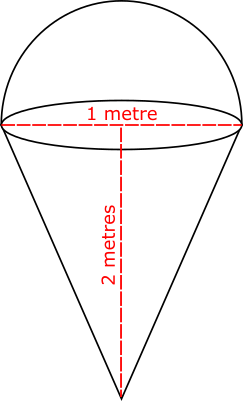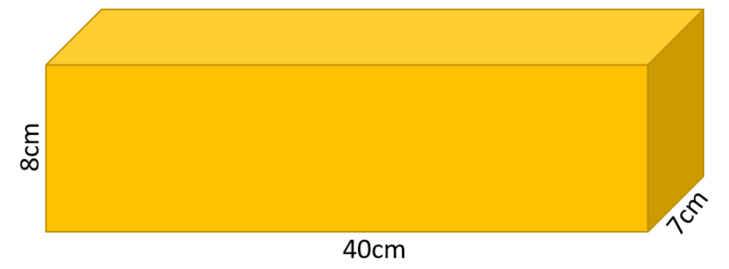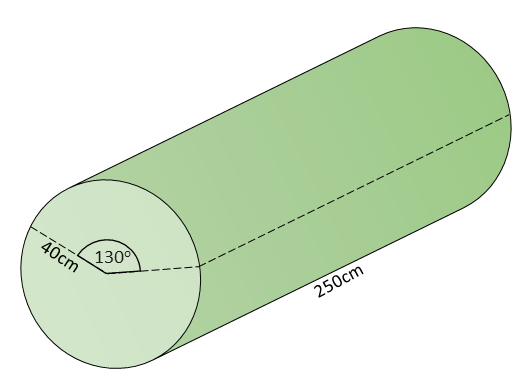# Exam-Style Questions on Volume

## Problems on Volume adapted from questions set in previous Mathematics exams.

### 1.

GCSE Higher

The diagram, not drawn to scale, shows the plan for a large, three dimensional sign which has been ordered to hang outside an ice cream shop.The sign is made of a hemisphere on top of a right cone.

The height of the cone is 2 m.

The top of the cone has a diameter of 1 m.

The hemisphere has a diameter of 1 m.

The total volume of the shape is $$k \pi$$ cm3, where $$k$$ is an integer.

Work out the value of k.

## Useful formulas

$$\text{Volume of a sphere} = \frac{4 \pi r^3}{3}$$

Where $$r$$ is the radius of the sphere.

$$\text{Volume of a cone} = \frac{\pi r^2 h}{3}$$

Where $$r$$ is the radius of the circular end of the cone and $$h$$ is the height of the cone.

### 2.

GCSE Higher

A container is in the shape of a cuboid as shown in the diagram below.

The container is three quarters full of water.

A glass holds 250 ml of water.

What is the greatest number of glasses that can be completely filled with water from the container?### 3.

GCSE Higher

The diagram shows a rectangular-based pyramid, TABCD (not drawn to scale).

The horizontal base ABCD has sides of lengths 11m and 15m. The centre of the base of the pyramid is M.

Angle TMC is 90° and angle TCM is 70°

The volume of a pyramid is $$\frac13$$ × area of base × perpendicular height. Calculate the volume of this pyramid.### 4.

IB Applications and Interpretation

A wedge is to be cut from a log in the shape of a cylinder as shown in the diagram below (not to scale).

The length of the log is 240cm and its radius is 40cm. The cross section of the wedge to be removed is a sector with an angle of 130o.

What is the volume of the remaining piece of the log after the wedge has been removed?If you would like space on the right of the question to write out the solution try this Thinning Feature. It will collapse the text into the left half of your screen but large diagrams will remain unchanged.

The exam-style questions appearing on this site are based on those set in previous examinations (or sample assessment papers for future examinations) by the major examination boards. The wording, diagrams and figures used in these questions have been changed from the originals so that students can have fresh, relevant problem solving practice even if they have previously worked through the related exam paper.

The solutions to the questions on this website are only available to those who have a Transum Subscription.

Exam-Style Questions Main Page

Search for exam-style questions containing a particular word or phrase:

To search the entire Transum website use the search box in the grey area below.